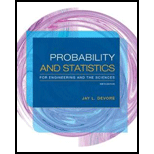# At a certain gas station, 40% of the customers use regular gas ( A 1 ), 35% use plus gas ( A 2 ), and 25% use premium ( A 3 ). Of those customers using regular gas, only 30% fill their tanks (event B ). Of those customers using plus, 60% fill their tanks, whereas of those using premium, 50% fill their tanks. a. What is the probability that the next customer will request plus gas and fill the tank ( A 2 ∩ B )? b. What is the probability that the next customer fills the tank? c. If the next customer fills the tank, what is the probability that regular gas is requested? Plus? Premium?### Probability and Statistics for Eng...

9th Edition
Jay L. Devore
Publisher: Cengage Learning
ISBN: 9781305251809### Probability and Statistics for Eng...

9th Edition
Jay L. Devore
Publisher: Cengage Learning
ISBN: 9781305251809

#### Solutions

Chapter
Section
Chapter 2.4, Problem 59E
Textbook Problem

## At a certain gas station, 40% of the customers use regular gas (A1), 35% use plus gas (A2), and 25% use premium (A3). Of those customers using regular gas, only 30% fill their tanks (event B). Of those customers using plus, 60% fill their tanks, whereas of those using premium, 50% fill their tanks. a. What is the probability that the next customer will request plus gas and fill the tank (A2 ∩ B)? b. What is the probability that the next customer fills the tank? c. If the next customer fills the tank, what is the probability that regular gas is requested? Plus? Premium?

Expert Solution

a.

To determine

Find the probability that the customer requests plus gas and fill the tank

### Explanation of Solution

Given info:

The data consists of three types of gas in the gas station and has two methods to fill their tanks. The probability of the customers using regular gas is 40%, the probability of the customers using plus gas is 35%, and the probability of the customers using premium gas is 25%.

30% of customers fill the gas given that they use regular gas. 60% of customers fill the gas given that they use plus gas and 50% of customers fill the gas given that they use premium gas.

Calculation:

Here, A represents customers using the gas, A1 represents the customers using regular gas, A2 represents the customers using plus gas, A3 represents the customers using premium gas, and B represents the customers fill the tanks

The probability that the customer requests regular gas and fill the tank is obtained as:

P(regular gas and fill the tank)=P(A1)P(B|A1)=0.4×0.3=0.12

The probability that the customer requests plus gas and fill the tank is obtained as:

P(plus gas and fill the tank)=P(A2)P(B|A2)=0

Expert Solution

b.

To determine

Find the probability that the customer fills the tank.

Expert Solution

c.

To determine

Find the probability that the customer requested regular gas.

Find the probability that the customer requested plus gas.

Find the probability that the customer requested premium gas.

### Want to see the full answer?

Check out a sample textbook solution.See solution

### Want to see this answer and more?

Bartleby provides explanations to thousands of textbook problems written by our experts, many with advanced degrees!

See solution